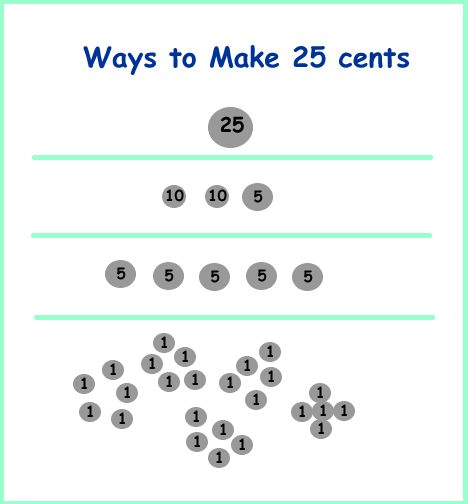# Different Ways to Communicate Mathematically

Mathematical ideas can be represent in multiple ways, six of the most common are with:

1. Concrete objects - example - Manipulatives use of - cubes Writing after
Average student height
2. Models - example - Cuisenaire models for multiplication of fractions
3. Pictures - example - How many ways to make 25 cents
4. Diagrams - example - Multiplication of two digit numbers related to the multiplication of binomals
5. Words (written and oral) - example - Percentage - Sale problem - What is the price of a pair of shoes on sale?
6. Act out - example - The Doorbell Rang by Pat Hutchins, sharing 12 cookies.
7. Symbols - example - Pigs Go to Dinner by Amy Axelrod, What did the family eat & what did it cost?

# The Doorbell Rang by Pat Hutchins - sharing 12 cookies (1986)

Read the book and ask the students to act out the story and list the different ways the cookies could have been shared as different numbers of children arrive.Hint:

Act out the Problem:

Use a tray and 12 objects (cookies). Put the 12 objects on a tray, read the book, ring a bell when you get to the doorbell ringing in the book and have a group of children equal to the number in the book move to the tray. Ask and record the number of cookies, the number of student,s and how many cookies each person would have. Continue through the book and record each step to solve the problem.

# Pigs Go to Dinner - What did the family eat & what did it cost?

After eating everything at home the Pigs decide to scavenger for money. They search the house and find loose change and bills enough to go for Mexican food at the Enchanted Enchilada. While there are some inaccuracies it is possible to calculate a value for the money found by the Pigs and a menu is provided to speculate what the Pigs may have feasted on.

The pigs found

1.00 + 2.00 + .10 + .05 + .25 + 5.00 + 1.00 + 1.00 + .50 + .17 + .60 + 1.00 + 2.00

I used a calculator and found the total was \$34.67

# What ways can a cashier make 25 cents?# Use cubes to measure a group of students & find their average height.

To find the average height of our group we measured all of us with multi link cubes. I measured 85.

We snapped them together and laid them all in a line.

Then we arranged them so they were all made the same height.

They were all 79 cubes tall.

We had left overs that we did not use, but there were not enough cubes to put one on each of the stacks that represented each student.

# What is the price of a pair of shoes on sale?

Sale 25% off! regularly \$80.00

The shoes would be \$60.00 without tax.

I figured they were \$80.00 not on sale and they were on sale for 25% off.

I thought of 25% as 1/4.

And 1/4 of \$80.00 was \$20.00

(20 + 20 +20 + 20 = 80)

(1/4 + 1/4 + 1/4 + 1/4 = 1)

so they must of cost \$60.00.

(20 + 20 + 20 = 60)